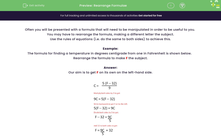# Rearrange Algebraic Formulae

In this worksheet, students will learn how to rearrange formulae to find the given letter (subject) of the formula.Key stage:  KS 3

Curriculum topic:   Algebra

Curriculum subtopic:   Simplify Algebraic Expressions to Maintain Equivalence

Difficulty level:#### Worksheet Overview

Often we will be presented with a formula that will need to be manipulated in order to be useful to us.

We may have to rearrange the formula, making a different letter the subject.

Use the rules of equations (i.e. do the same to both sides) to achieve this.

Example:

The formula for finding a temperature in degrees centigrade from one in Fahrenheit is shown below.

Rearrange the formula to make F the subject.

Our aim is to get F on its own on the left-hand side.And we're there!!

Now it's over to you.

### What is EdPlace?

We're your National Curriculum aligned online education content provider helping each child succeed in English, maths and science from year 1 to GCSE. With an EdPlace account you’ll be able to track and measure progress, helping each child achieve their best. We build confidence and attainment by personalising each child’s learning at a level that suits them.

Get started